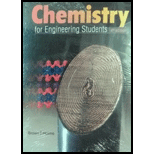Chapter 5, Problem 5.90PAEChemistry for Engineering Students

4th Edition
Lawrence S. Brown + 1 other
ISBN: 9781337398909Chemistry for Engineering Students

4th Edition
Lawrence S. Brown + 1 other
ISBN: 9781337398909
Textbook Problem

90 You work in a semiconductor production plant that relies on several chlorofluorocarbons in its manufacturing process. One day, you find an unlabeled gas cylinder, and you are assigned to figure out what is in the tank. First, you fill a 1.000-L fla1k with the gas. At a pressure of 250.0 torr and a temperature of 25.00°C, you determine that the mass of the gas in the flask is 2.2980 g. Then, you send the flask to an outside lab for elemental analysis, and they report that the gas contains 14.05% C, 44.46% F, and 41.48% Cl by mass. Find the molecular formula of this gas.

Interpretation Introduction

Interpretation:

You work in a semiconductor production plant that relies on several chlorofluorocarbons in its manufacturing process. One day, you find an unlabeled gas cylinder, and you are assigned to figure out what is in the tank. First, you fill a 1.000-L flask with the gas. At a pressure of 250.0 torr and a temperature of 25.000C, you determine that the mass of the gas in the flask is 2.2980 g. Then, you send the flask to an outside lab for elemental analysis, and they report that the gas contains 14.05% C, 44.46% F, and 41.48% Cl by mass. The molecular formula of this gas should be identified.

Concept introduction:

An ideal gas which is known as the perfect gas is a gas whose volume V, Pressure P and temperature T are related through the ideal gas laws: -

PV = nRT

Here,

n = Number of moles of the gas

R = Universal gas constant

T = Temperature

P = Pressure

Ideal gases are described as the molecules which have negligible size but have an average molar kinetic energy which is dependent on the temperature. When temperature is low most of the gases behave like ideal gases and the ideal gas law might be applied to them.

Explanation

The ideal gas equation is as follows:

PV=nRT

Or,

PV=(mM) RT

Or,

M=mRT/PV

=( 2.298 g)( 0.0821 atm L/mol K)( 298 K)( 0.329 atm)( 1 L)=170.88 g/mol

Now, the percent composition of the given elements are:

C=14.05%F=44.46%Cl=41.48%

Divide all with their molar masses to get the number of atoms of each element as follows

C =  14.02 12 = 1.17F =  44.46 18.9  = 2.35Cl =  41.48 35.5 = 1.17

Now, divide with the smallest number to calculate the empirical formula:

C =  1.17 1.17 =1 F =  2

Still sussing out bartleby?

Check out a sample textbook solution.

See a sample solution

The Solution to Your Study Problems

Bartleby provides explanations to thousands of textbook problems written by our experts, many with advanced degrees!

Get Started